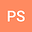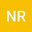CMMSE: POWERS OF CATALAN GENERATING FUNCTIONS FOR BOUNDED OPERATORS
••## Abstract

In this paper we consider the Catalan triangle numbers ( B n , k ) n ≥ 1 , 1 ≤ k ≤ n and ( A n , k ) n ≥ 1 , 1 ≤ k ≤ n + 1 to define powers of Catalan generating function C( T) where T is a linear and bounded operator on a Banach space X. When the operator 4 T is of power-bounded operator, the Catalan generating function is given by the Taylor series C ( T ) : = ∑ n = 0 ∞ C n T n , where c = ( C n ) n ≥ 0 is the Catalan sequence. Note that the operator C( T) is a solution of the quadratic equation T Y 2 − Y + I = 0 . We obtain new formulae which involves Catalan triangle numbers ( B n , k ) n ≥ 1 , 1 ≤ k ≤ n and ( A n , k ) n ≥ 1 , 1 ≤ k ≤ n + 1 . As element in the Banach algebra ℓ 1 ( N 0 , 1 4 n ) , we describe the spectrum of c ∗ j for j≥1, and the expression of c −∗ j in terms of Catalan polynomials. In the last section, we give some particular examples to illustrate our results and some ideas to continue this research in the future.
16 Sep 2022Submitted to Mathematical Methods in the Applied Sciences
16 Sep 2022Submission Checks Completed
16 Sep 2022Assigned to Editor
22 Sep 2022Reviewer(s) Assigned
30 Jan 2023Review(s) Completed, Editorial Evaluation Pending
10 Feb 2023Editorial Decision: Revise Major